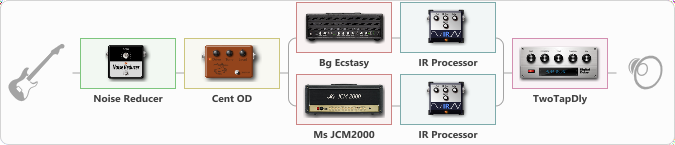# Ritmica

Discussion in 'ToneLib-GFX presets' started by carlinos3, Feb 13, 2020.

1. Ritmica

Preset name: Tapping Monster

Effects chain:Effect: "Noise Reducer" (Dynamics / Filter), active - "yes"
{
"Sens" = 52
"Mode" = Hard
}

Effect: "Cent OD" (Overdrive / Distortion), active - "yes"
{
"Drive" = 27
"Tone" = 77
"Level" = 38
}

Effect: "Splitter" (Dynamics / Filter), active - "yes"
{
"A-Bypass" = Off
"A-Pan" = -100
"A-Level" = 55
"B-Bypass" = Off
"B-Pan" = 100
"B-Level" = 55

Left branch:
{

Effect: "Bg Ecstasy" (Amp simulators), active - "yes"
{
"Gain" = 100
"Bass" = 50
"Middle" = 68
"Treble" = 74
"Presence" = 50
"Master" = 76
"Level (dB)" = 6
}

Effect: "IR Processor" (Cabinets), active - "yes"
{
"IR" = charv.1_dc
"Low Cut (Hz)" = 0
"Hi Cut (kHz)" = 20.0
"Mix" = 100
"Level (dB)" = 4
}
}
Right branch:
{

Effect: "Ms JCM2000" (Amp simulators), active - "yes"
{
"Gain" = 100
"Bass" = 50
"Middle" = 50
"Treble" = 50
"Presence" = 86
"Master" = 64
"Level (dB)" = 12
}

Effect: "IR Processor" (Cabinets), active - "yes"
{
"IR" = charv.1_dc
"Low Cut (Hz)" = 0
"Hi Cut (kHz)" = 20.0
"Mix" = 100
"Level (dB)" = 0
}
}
}

Effect: "TwoTapDly" (Delay), active - "yes"
{
"Time" = 564
"Feedback" = 0
"Tone" = 77
"Sens" = 0
"TapTime" = 5.0
"Mix" = 43
}

Note: You will need to download and install the ToneLib-GFX software to use the preset.

#### Attached Files:

• ###### Ritmica.tlgfx
File size:
117.7 KB
Views:
4,040
Solo, SEMENOV, Grisha and 6 others like this.